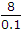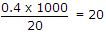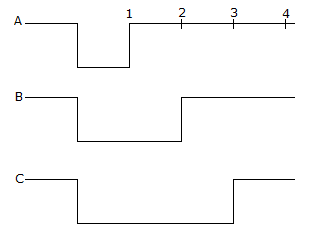# Electronics and Communication Engineering - Digital Electronics

41.

TTL is used in electronic calculators.

 A. True B. False

Explanation:

Calculators use CMOS.

42.

Inverter 74 LS04 has following specifications I0H max = - 0.4 mA, I0L max = 8 mA, IIH max = 20 μA, IIL max = 0.1 mA
The fan out of this inverter is

 A. 10 B. 20 C. 60 D. 100

Explanation:

Fan out in low state == 80 Fan out in high state =Hence fan out is smaller of two values ie., 20.

43.

A 4 bit ripple counter starts in 0000 state. When the counter reads 0010 the number of clock pulses which have occurred is

 A. 2 B. 18 C. 2 or 18 D. 2 or 18 or 34

Explanation:

At pulse 1 the state changes to 0001 and at pulse 2 it changes to 0010 Since it has a total of 16 states, the counter returns to 0000 after 16 and 32 pulses.

44.

The number FF in hexadecimal system is equivalent to number __________ in decimal system.

 A. 256 B. 255 C. 240 D. 239

Explanation:

FF = 15 x 16 + 15 = 255.

45.

The inputs A, B, C of the given figure are applied to a 3 input NOR gate. The output isA. HIGH from 4 to 0 B. LOW from 0 to 4 C. HIGH from 0 to 1 and LOW from 1 to 4 D. LOW from 0 to 2 and HIGH from 2 to 4